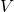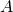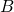This is the fifty fourth part of the ILP series. For your convenience you can find other parts in the table of contents in Part 1 – Boolean algebra

Last time we saw how to implement max flow in ILP. Today we are going to use this approach to make sure that the graph is connected.

First, we create a new vertex representing the supersource of a graph. We connect this vertex to any of the existing verticesand set edge capacity to infinity.

Next, we create a new vertex representing the supersink. We connect every vertex of the original graph with the supersink. We set each edge capacity to one.

Finally, we calculate the flow formula but this time we set it to the number of vertices in the original graph. Thanks to that we make sure that all vertices are reachable from.

This way we defined the connected component (not necessarily strongly connected component). We can modify this approach slightly to ensure that a given pair of verticesandis connected. Connect supersource to, connect onlyto supersink and require the flow equal to one.